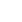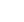• English
• Hindi
• Bengali
• Marathi
• Telugu
• Tamil
• Gujarati
• Odia
• Malayalam

## Commercial Vehicle Loan EMI Calculator

Calculating your Commercial Vehicle Loan EMI has never been easier. Use our Commercial Vehicle Loan EMI Calculator to input your desired amount, interest rate and tenure, and view an instant summary of your EMI amounts. You can also simply adjust the amount and tenure to see how it affects your EMI repayments. Get on the road faster with our Commercial Vehicle Loan EMI Calculator.

1L 1Cr
6% 26%
12 60
Total Amount Payable

• Principal Amount

• Total Amount

Monthly EMI

### Commercial Vehicle Loan EMI: What Is It?

A Commercial Vehicle Loan EMI (Equated Monthly Instalment) is the monthly payment you make until your vehicle loan is fully repaid. This amount comprises both the principal loan amount and the interest accrued.

### Commercial Vehicle Loan EMI: How Is It Calculated?

To calculate your Commercial Vehicle Loan EMI manually, you can use this formula:

EMI = [P x R x (1+R)^N]/[(1+R)^N-1]

• P represents the loan amount.
• R is the monthly interest rate (annual interest rate divided by 12).
• N denotes the loan tenure in months.

For example, if you take a commercial vehicle loan of ₹10,00,000 at an interest rate of 10% per annum for a term of 5 years, your monthly EMI would be:

EMI = [1000000 x 0.1/12 x (1+0)]

EMI = ₹8333.33

### Benefits of Using a Commercial Vehicle Loan EMI Calculator

• Speed and convenience: It’s a quick and easy tool.
• Accuracy: Ensures precise EMI calculations.
• Financial planning: Helps you budget for your commercial vehicle

### How to Use Mahindra Finance Commercial Vehicle Loan EMI Calculator?

Using a commercial vehicle finance EMI calculator is simple. Just provide three key inputs:

• Loan
• amount-Interest
• rate-Loan tenure

The calculator will then display your monthly EMI. Feel free to experiment with different loan amounts, interest rates, and tenures to find the most suitable option for your budget.